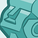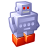# QlikView App Dev

Discussion Board for collaboration related to QlikView App Development.

Announcements
Now Live: Qlik Sense SaaS Simplified Authoring – Analytics Creation for Everyone: READ DETAILS
cancel
Showing results for
Did you mean:Contributor III

## Total Average of Averages

I have a pivot table with two dimensions. I have an expression that calculates average in a field, I found that using avg(Field) doesn't give me the correct subtotal/total by dimension (using show partial sums). I am using the expression:

avg(aggr(avg(Field), Dim1, Dim2))

I get the correct subtotal (Dim1) but the total (Dim2) is less than it should be.

Any help will be appreciated.  Thanks

1 Solution

Accepted SolutionsMVP

May be this

Avg(Aggr(Avg(Aggr(Avg({<Dim2 = {"=Avg(FIELD) > 0"}>}FIELD), DIM1, DIM2)), DIM2))

26 RepliesCreator

try this sum(aggr(avg( field), dim1, dim2 .....))Contributor III
Author

That gives me a total of the averages. I am looking for an average of the averages. For example your result gave me the total of my averages at 235.95 for dim1 what I want is that divided by the count of the dim1 (7) for a result of 33.71. Dim1 result is fine, it's dim2 total I'm not getting. It should be sum of subtotals for dim1 divided by count of dim 2.

Thanks for the suggestions.Creator

can you provide some sample dataContributor III
Author
 Dim2 Dim1 Avg TAT A 22.80 B 31.11 C 7.98 D 5.36 E 1.38 F 7.72 G 1 6.50 G 2 21.74 G 3 5.50 G 4 147.73 G 5 32.83 G 6 16.72 G 7 4.93 G Total 33.71 Correct H 32.21 I 18.29 J 22.12 Total 23.15 Incorrect

Here you goCreator

try this AVG(AGGR(AVG(aggr(avg(FIELD),DIM1,DIM2)),DIM2))Contributor III
Author

Getting close. Sum of subtotal averages is 182.68. Count of dim2 is 10. I should be getting total average of 18.27.

ThanksCreator

what is the answer are you getting now ?Contributor III
Author

16.61Creator II

I would suggest use dimensionality to control what is displayed  in the subtotal and total fields, eg

if (dimensionality()=1, aggr(avg()), avg())